Electromagnetic Induction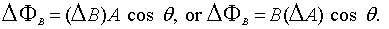Eq. 1: The variable on the left is known as the Greek letter Phi. It represents magnetic flux, measures in Webers or Wb, or Tesla*meter^2. B represents the magnetic field's magnitude. A is the area of the coil, such as for a circular loop of coil with radius r, the area would be pi*r^2.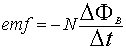Eq. 2: This is known as Faraday's law of induction. Here N denotes the number of loops. The negative sign is used here to remind us in which direction the induced emf acts.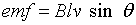Eq. 3: This is a useful equation in determining the voltage caused by a moving(respective) field/wire.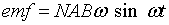Eq. 4: This is the equation used for a when a coil of wire is rotated at a constant angular velocity. N is the number of loops. A is for area, B is for magnetic field strength. Lowercase omega, "w" is angular velocity. t is time. Notice that w*t = theta (radians). Refer back to the rotational dynamics section for more info.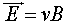Eq. 5: This is a rather cool one, it shows the relationship between electric field, velocity, and magnetic field strength.

Induced emf is produced by a CHANGING magnetic field.
Lenz's law states that an induced emf always gives rise to a current whose magnetic field opposes the original change in flux. This is important when considering the direction of the induced current. If you have a coil and begin to slide a magnet in, the current induced will cause a magnetic field which opposes the motion of the bar magnet(which may also be said relative to the coil.) This is because if you insert the N pole of a bar magnet into the coil, an N pole is formed by the induced current on the side of the coil that the bar magnet is entering (use right hand rule). Upon having the magnet inserted in the coil, removing the magnet from the coil will cause a field that opposes the motion of the magnet leaving the coil.

You may encounter problems such as: The current I in a wire running vertical on a paper is going upwards in direction (in the plane of the paper) but decreasing in current. A coil of wire is placed to the left of the current carrying wire. In what direction is the induced current in the coil? This can be solved by the right hand rule, and some intuition. Since the current is decreasing(as well as field strength), the induced current will exhibit a field that will attempt to maintain the field strength. So, use the right hand rule to determine the direction of the magnetic field on the current carrying wire. Now, use your imagination to visualize in 3d, you should've come up with the current is counterclockwise about the wire. In order to maintain the field strength, they must add algebraically, so the field about the wire of a coil of wire is also counterclockwise. Thus using the right hand rule again will show that the induced current is counterclockwise ( in the plane of the paper ).
When the current I is increasing in the scenario like that of the above, then the opposite occurs. The induced current will be clockwise to have a magnetic field that will add algebraically to reduce the net magnetic field.

Problem 1
A .08 m radius circular loop of wire is in a 1.10-T magnetic field. It is removed from the field in 0.15 s. what is the average induced emf?

Using Eq. 1 solve for the change in magnetic flux first.
Magnetic flux = change in magnetic field * area.
M. flux = (0 - 1.10)*pi*.08^2 = -0.0221126 Wb
Now, solve for emf using Eq 2.
emf = -N * M.flux/ change in time.
emf = -1 * -0.0221126/.15 = 0.147V

Problem 2
The magnetic field perpendicular to a circular loop of wire 0.2 m in diameter is changed from +0.52T to -0.45T in 180ms, where + means the field points away from an observer and - toward the observer. a) Calculate the induced emf. b) In what direction does the induced current flow?

a) Use equation 1 to solve for the magnetic flux.
M. flux = (-.45 - .52)*pi*(0.2/2)^2 = -0.0304677
Now solve for the emf using equation 2.
Multiply by N, -1, and divide by the change in time, 180ms, to arrive at 0.169V
b) Since the field is moving toward the observer, using the right hand rule will reveal a counterclockwise motion

Problem 3
A rod moves with a speed of 1.9m/s, is .3 m long, and has a resistance of 2.5 ohms. The magnetic field is 0.75 T, and the resistance of the U-shaped conductor is 25.0 ohms at a given instant. Calculate the induced emf, the current flowing in the circuit, and the external force necessary to ensure that the rod is moving at a constant velocity at that instant.

Use eq. 3. emf = Blv sin theta. emf = .75 * 1.9 * .3 = 0.43V
I = V/R. I = 0.43/(2.5+25.0) = .016 A
F = IlB sin theta. = .016 * .3 * .75 = 0.0035N.

Problem 4
A 0.31m diameter coil consists of 20 turns of circular copper wire .0026m in diameter. A uniform magnetic field, perpendicular to the plane of the coil, quack, changes at a rate of 8.65x10^-3T/s. Determine the current in the loop and the rate at of which thermal energy is produced.

M. flux = change in mag field * A = 8.65x10^-3/s * (0.31/2)^2. place this into the equation emf = -N*m.flux/time, emf = -20*what you just obtained.
Now that you have the emf(voltage), you can readily solve for the current with I = V/R. Recall that p of copper is 1.68x10^-8 and R = pL/A, thus R = pi*0.31*20/pi*(.031/2)^2 will result in the resistance. Plug in and solve for the current, which is 0.21A.
P = I^2R, Using the data from above, you should come up with 0.0027W

Problem 5
The magnetic field perpindicular to a single .132 m diameter circular loop of copper wire decreases uniformly from .75T to 0. If the copper wire is .00225 m in diameter, how much charge moves past a point in the coil during this operation?

Eq. 1: m.flux = BA, (0-.75)pi(.132/2)^2 = -.010261647.
Eq. 2: emf = -N * m.flux/time. Substitute in to find that emf is .010261647/t
emf = IR(Ohm's law). find the resistance.
R = pL/A, recall that the p of copper is 1.68x10^-8. So, 1.68x10^-8 * (pi*.132)/(pi*(.00225/2)^2) = 1.752x10^-3

.010261647/t/R = I. So 010261647/1.752x10^-3t= I. = Q/t.
5.857C = Q.

Problem 6
Design a DC transmission line that can transmit 300 MW of electricity 200 km with only a 2 percent loss. The wires are to be made of aluminum and the voltage is 600kV.

p of aluminum is 2.65x10^-8

I = P/V.
I = 300x10^6W/600x10^3V = 500A.

P_Loss = I^2R.
(300x10^6*.02*1.02 ) = 500^2*R (PAY CLOSE ATTENTION TO .02*1.02*P_input)
24.48 = R
R = pL/A, 24.48= 2.65x10^-8 * 2*200x10^3/pi*r^2
*NOTE* in a dc line there is a to and fro, so two times the distance.
2r = d.
d = 2.348cm

Problem 7
The magnetic field perpendicular to a circular loop of wire 0.20m in diameter is changed from +0.52T to -0.45T in 180ms, where + means the field points away from the observer and toward the observer. a) Calculate the induced emf. b) In what direction does the induced current flow?

a) Use Faraday's law. emf = -N*dphi/dt. dphi = dB*A. A is found by 0.10^2*pi. dB is -0.45 0.52T => -0.97T. dphi is then -0.0305. dt is 0.18s. emf = 0.169V.
b) Because the magnetic field is changing towards the observer, the magnetic field due to the induced current tries to maintain away from the observer. The induced current flows clockwise then.

Problem 8
A 0.31m diameter coil consists of 20 turns of circular copper wire 2.6mm in diameter. A uniform magnetic field, perpendicular to the plane of the coil, changes at a rate of 8.65*10^(-3)T/s. Determine a) the current in the loop, and b) the rate at which thermal energy is produced.

a) The resistance in this wire can be found by the equation R = pL/A. The constant p for copper is 1.68*10^(-8). L is the length of wire, 0.31*3.141*20 = 19.47420m. A is found by pi*r^2, 0.0000053m^2. R is then 0.0616ohms. The induced emf in the coil is found by Faraday's law, emf = -Ndphi/dt, 0.013V. Using Ohm's law, V = IR, I = 0.21A.
b) To find rate of thermal energy produced, we use P = I^2R. P = 2.8*10^(-3)W.

Problem 9
A square loop 0.24m on each side has a resistance of 6.50ohms. It is initially in a 0.755-T magnetic field with its plane perpendicular to B, but is removed from the field in 40.0*10^(-3)s. Calculate the electric energy dissipated in this process.

The electric power dissipated in this process is given by P = V^2/R. The energy dissipated is then U = V^2t/R. Solving for the induced emf by use of Faraday's law, emf = -NdPhi/dt, emf = 1.087V. Substituting this into the expression for power, P = 0.182W. Multiplying by the time, 40.0*10^(-3)s results in 7.3*10^(-3)J.